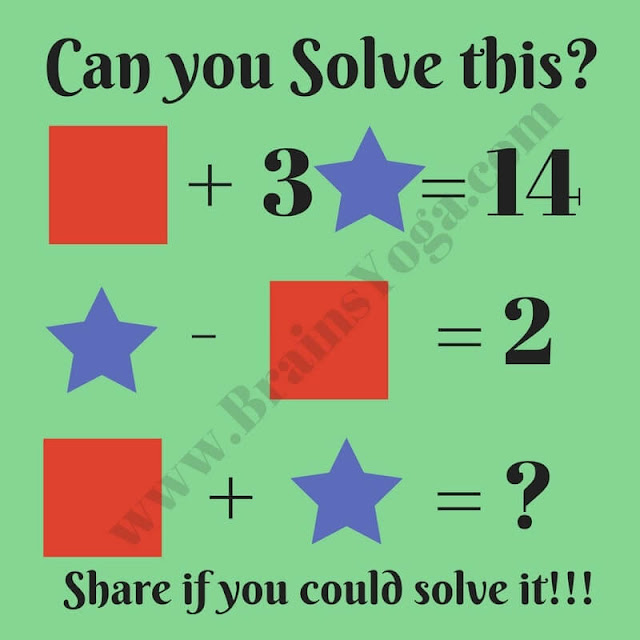Mathematics is a fun subject. Many Maths problems can be put in the form of a puzzle image. Here is one maths puzzle in this picture image. Your challenge in this puzzle is to decode the values of the different symbols shown in the puzzle image. Once you are able to decode their values, find the value of the missing number in the last equation. Can you solve this Maths Picture Puzzle?Mathematical Picture Riddle

#### 1 comment:

Anonymous said...